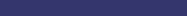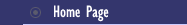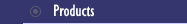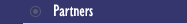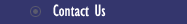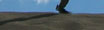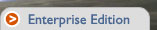Home > Products > McCabe IQ > Research Library > Software Metrics Glossary

Software Metrics Glossary

McCabe IQ uses a vast number of software metrics to get the most precise assessment of your application's quality, security, and testing. We've defined these metrics below for your reference. Further details on many of these metrics can be found in the NIST document- "Structured Testing: A Testing Methodology Using the Cyclomatic Complexity Metric" by Arthur Watson and Tom McCabe.

 McCabe Metrics Cyclomatic Complexity Metric (v(G)) Cyclomatic Complexity (v(G)) is a measure of the complexity of a module's decision structure. It is the number of linearly independent paths and therefore, the minimum number of paths that should be tested. Actual Complexity Metric (ac) Actual Complexity (ac) is the number of independent paths traversed during testing. Module Design Complexity Metric (iv(G)) Module Design Complexity (iv(G)) is the complexity of the design-reduced module and reflects the complexity of the module's calling patterns to its immediate subordinate modules. This metric differentiates between modules which will seriously complicate the design of any program they are part of and modules which simply contain complex computational logic. It is the basis upon which program design and integration complexities (S0 and S1) are calculated. Essential Complexity Metric (ev(G)) Essential Complexity (ev(G)) is a measure of the degree to which a module contains unstructured constructs. This metric measures the degree of structuredness and the quality of the code. It is used to predict the maintenance effort and to help in the modularization process. Pathological Complexity Metric (pv(G)) pv(G) is a measure of the degree to which a module contains extremely unstructured constructs. Design Complexity Metric (S0) S0 measures the amount of interaction between modules in a system. Integration Complexity Metric (S1) S1 measures the amount of integration testing necessary to guard against errors. Object Integration Complexity Metric (OS1) OS1 quantifies the number of tests necessary to fully integrate an object or class into an OO system. Global Data Complexity Metric (gdv(G)) gdv(G) quantifies the cyclomatic complexity of a module's structure as it relates to global/parameter data. It can be no less than one and no more than the cyclomatic complexity of the original flowgraph. McCabe Data-Related Software Metrics Data Complexity Metric (DV) Data Complexity Metric (DV) quantifies the complexity of a module's structure as it relates to data-related variables. It is the number of independent paths through data logic, and therefore, a measure of the testing effort with respect to data-related variables. Tested Data Complexity Metric (TDV ) Tested Data Complexity Metric (TDV) quantifies the complexity of a module's structure as it relates to data-related variables. It is the number of independent paths through data logic that have been tested. Data Reference Metric (DR ) Data Reference Metric (DR ) measures references to data-related variables independently of control flow. It is the total number of times that data-related variables are used in a module. Tested Data Reference Metric (TDR ) Tested Data Reference Metric (TDR ) is the total number of tested references to data-related variables. Maintenance Severity Metric (maint_severity) Maintenance Severity Metric (maint_severity) measures how difficult it is to maintain a module. Data Reference Severity Metric (DR_severity) Data Reference Severity Metric (DR_severity) measures the level of data intensity within a module. It is an indicator of high levels of data related code; therefore, a module is data intense if it contains a large number of data-related variables. Data Complexity Severity Metric (DV_severity) Data Complexity Severity Metric (DV_severity ) measures the level of data density within a module. It is an indicator of high levels of data logic in test paths; therefore, a module is data dense if it contains data-related variables in a large proportion of its structures. Global Data Severity Metric (gdv_severity) Global Data Severity Metric (gdv_severity) measures the potential impact of testing data-related basis paths across modules. It is based on global data test paths. McCabe Object-Oriented Software Metrics ENCAPSULATION Percent Public Data (PCTPUB) PCTPUB is the percentage of PUBLIC and PROTECTED data within a class. Access to Public Data (PUBDATA) PUBDATA indicates the number of accesses to PUBLIC and PROTECTED data. POLYMORPHISM Percent of Unoverloaded Calls (PCTCALL) PCTCALL is the number of non-overloaded calls in a system. Number of Roots (ROOTCNT) ROOTCNT is the total number of class hierarchy roots within a program. Fan-in (FANIN) FANIN is the number of classes from which a class is derived. QUALITY Maximum v(G) (MAXV) MAXV is the maximum cyclomatic complexity value for any single method within a class. Maximum ev(G) (MAXEV) MAXEV is the maximum essential complexity value for any single method within a class. Hierarchy Quality(QUAL) QUAL counts the number of classes within a system that are dependent upon their descendants. OTHER Depth (DEPTH) DEPTH indicates at what level a class is located within its class hierarchy. Lack of Cohesion of Methods (LOCM) LOCM is a measure of how the methods of a class interact with the data in a class. Number of Children (NOC) NOC is the number of classes that are derived directly from a specified class. Response For a Class (RFC) RFC is a count of methods implemented within a class plus the number of methods accessible to an object of this class type due to inheritance. Weighted Methods Per Class (WMC) WMC is a count of methods implemented within a class. Halstead Software Metrics Program Length The total number of operator occurrences and the total number of operand occurrences. Program Volume The minimum number of bits required for coding the program. Program Level and Program Difficulty Measure the program's ability to be comprehended. Intelligent Content Shows the complexity of a given algorithm independent of the language used to express the algorithm. Programming Effort The estimated mental effort required to develop the program. Error Estimate Calculates the number of errors in a program. Programming Time The estimated amount of time to implement an algorithm. Line Count Software Metrics Lines of Code Lines of Comment Lines of Mixed Code and Comments Lines Left Blank